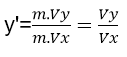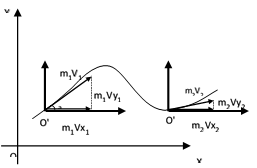Select Page

# Moving origins of coordinates

In the moving (object) origin of coordinates, 2 algebraic relations, 2 differential equations can be compared with each other

#### Physics , Mechanic , Mathematic

Introduction

In basic sciences such as math , physics , mechanics … and in order to show a relation or an equation , it is always shown or established on the static or fixed origin of coordinates . Regarding the print of the paper , “Application of Differential Equations in space” , in your periodical , it is shown that the moving object moves on differential equations , moving constantly on the fixed origin of coordinates in which case the movement of the moving object on differential equations in space does not depend on the mass of the moving object